Picture by Melanie Kreutz, Unsplash.

Categorical data can be visualised in many ways, and there’s no requirement to stick to the standard bar chart. Here are a selection of attractive Seaborn charts, graphs, and plots you can use to visualise and interpret categorical data in Pandas.

We only need a few packages for this project - Pandas for loading and viewing the text data, Numpy for some mathematical functions, and the Seaborn data visualisation package. Seaborn is built on top of Matplotlib and provides a quicker and easier way to create attractive looking charts and graphs. To make the images look sharper on high resolution displays, we’ll also set them to a larger figure size and enable “retina mode”.

``````import pandas as pd
import numpy as np
import seaborn as sns
``````
``````%config InlineBackend.figure_format = 'retina'
sns.set_context('notebook')
sns.set(rc={'figure.figsize':(15, 6)})
``````

You can use any data that contains categorical variables. The dataset I’m using is the Marketing Promotion Campaign Uplift Modelling dataset which is available from Kaggle. This looks to be a synthetic derivative of Kevin Hillstrom’s MineThatData dataset and is typical of the sort of thing you’ll encounter if you work in marketing or ecommerce. There are three categorical columns and a number of numeric ones.

``````df = pd.read_csv('data.csv')
``````
recency history used_discount used_bogo zip_code is_referral channel offer conversion
0 10 142.44 1 0 Surburban 0 Phone Buy One Get One 0
1 6 329.08 1 1 Rural 1 Web No Offer 0
2 7 180.65 0 1 Surburban 1 Web Buy One Get One 0
3 9 675.83 1 0 Rural 1 Web Discount 0
4 2 45.34 1 0 Urban 0 Web Buy One Get One 0

### Bar plots

Bar plots or bar charts are one of the most commonly used visualisations for categorical data. They can be created with a number of different Seaborn functions, but `barplot()` is the most common. They’re a good way to display the mean, sum, or count of values across the unique categorical values of a column. All derivatives of the Seaborn `barplot()` require the minimum of an `x` and `y` column name from a Pandas dataframe, plus the name of the dataframe.

#### Sum bar plots

To create a sum bar plot you pass in the categorical column name to `x` and the numeric column name to `y`, define the dataframe to use in the `data` argument and set the `estimator` argument to `sum`. On this dataset, the code below calculates the total spend for customers by channel.

``````sns.barplot(x="channel", y="history", data=df, estimator=sum, palette="husl")
``````
``````<matplotlib.axes._subplots.AxesSubplot at 0x7fb9ea761a30>
``````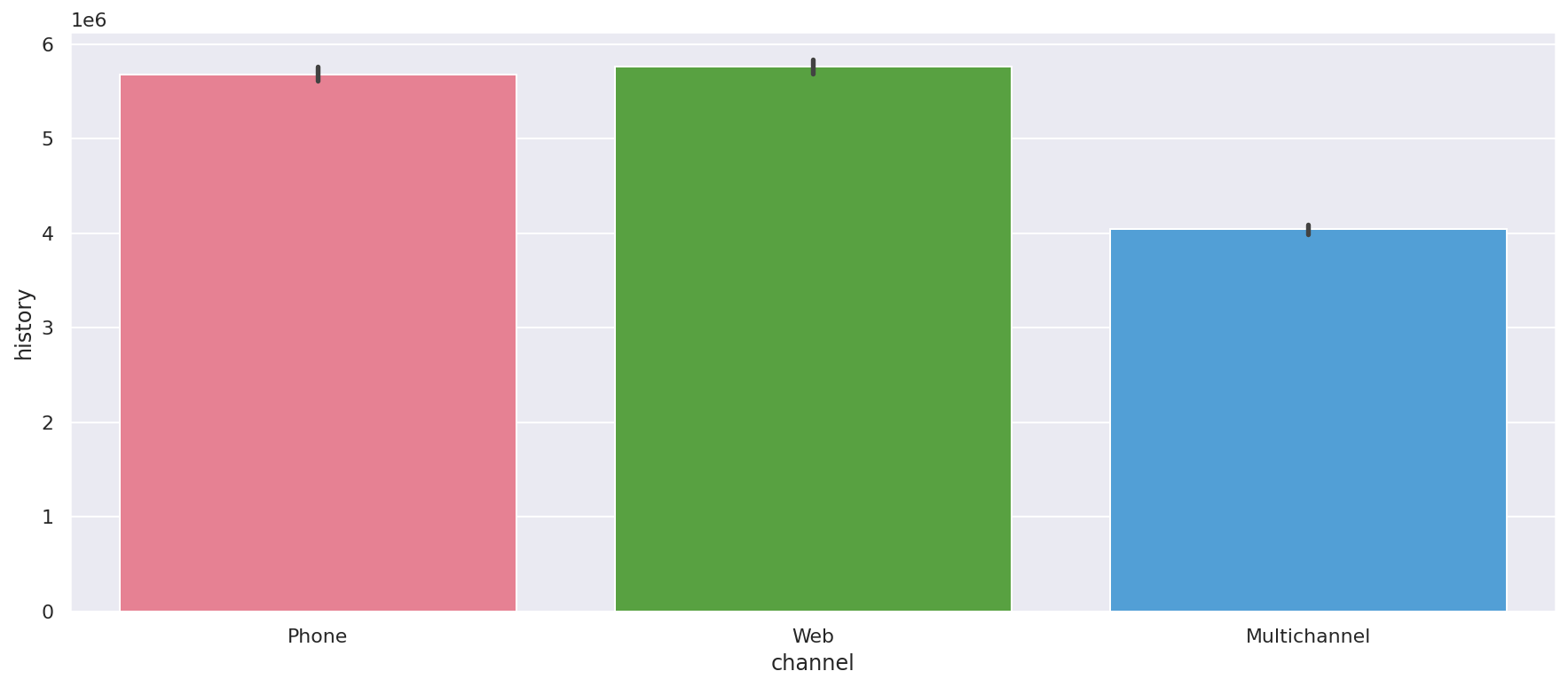#### Mean bar plots

You can pass any Numpy mathematical operator to the `estimator` argument, so to create a mean bar plot showing the mean spend by channel you can change `estimator=sum` to `estimator=np.mean`.

``````sns.barplot(x="channel", y="history", data=df, estimator=np.mean, palette="husl")
``````
``````<matplotlib.axes._subplots.AxesSubplot at 0x7fb9f81fc730>
``````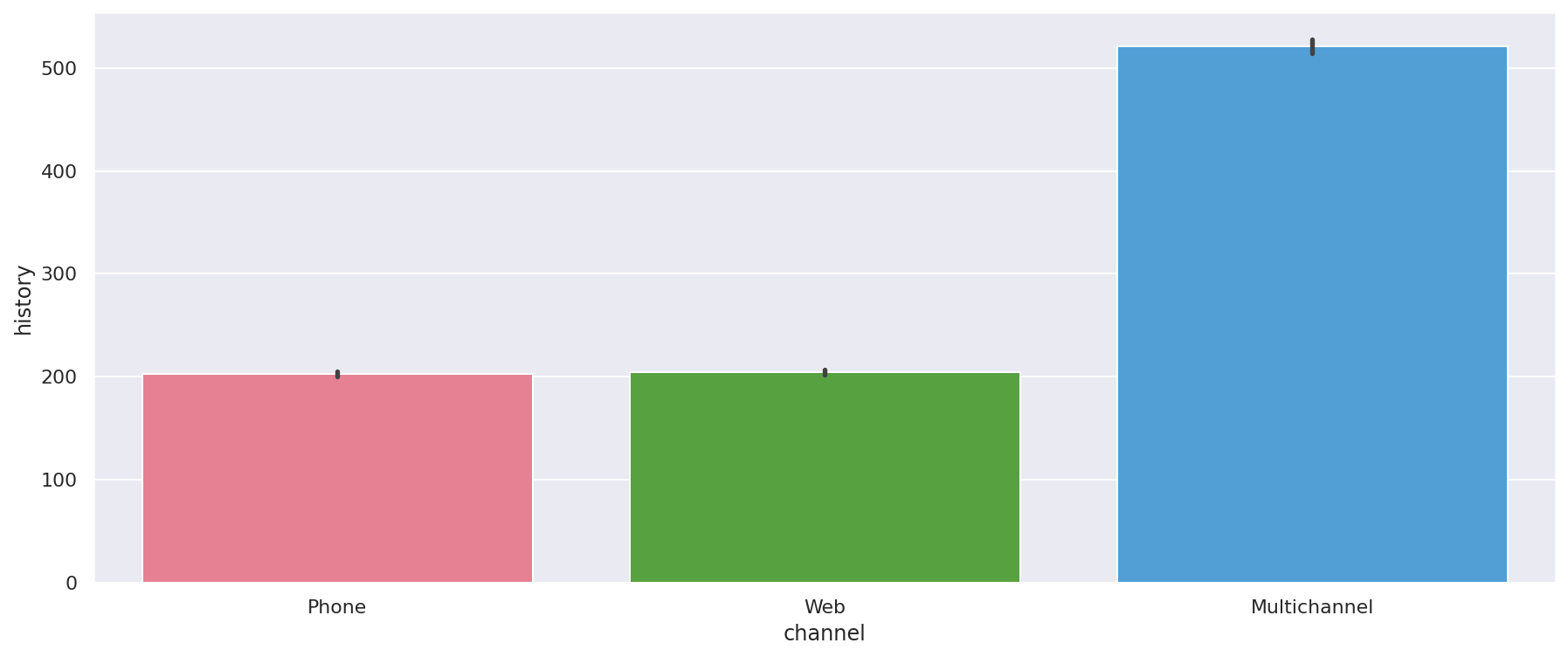### Count plots

Unlike bar plots, which take two values - an `x` and a `y` column - a count plot takes a single column value. For each unique categorical value found in the column, the count plot will display the count of items found. Here’s an example which counts the number of customers by `zip_code`.

``````sns.countplot(y="zip_code", data=df, palette="husl")
``````
``````<matplotlib.axes._subplots.AxesSubplot at 0x7fb9ea792df0>
``````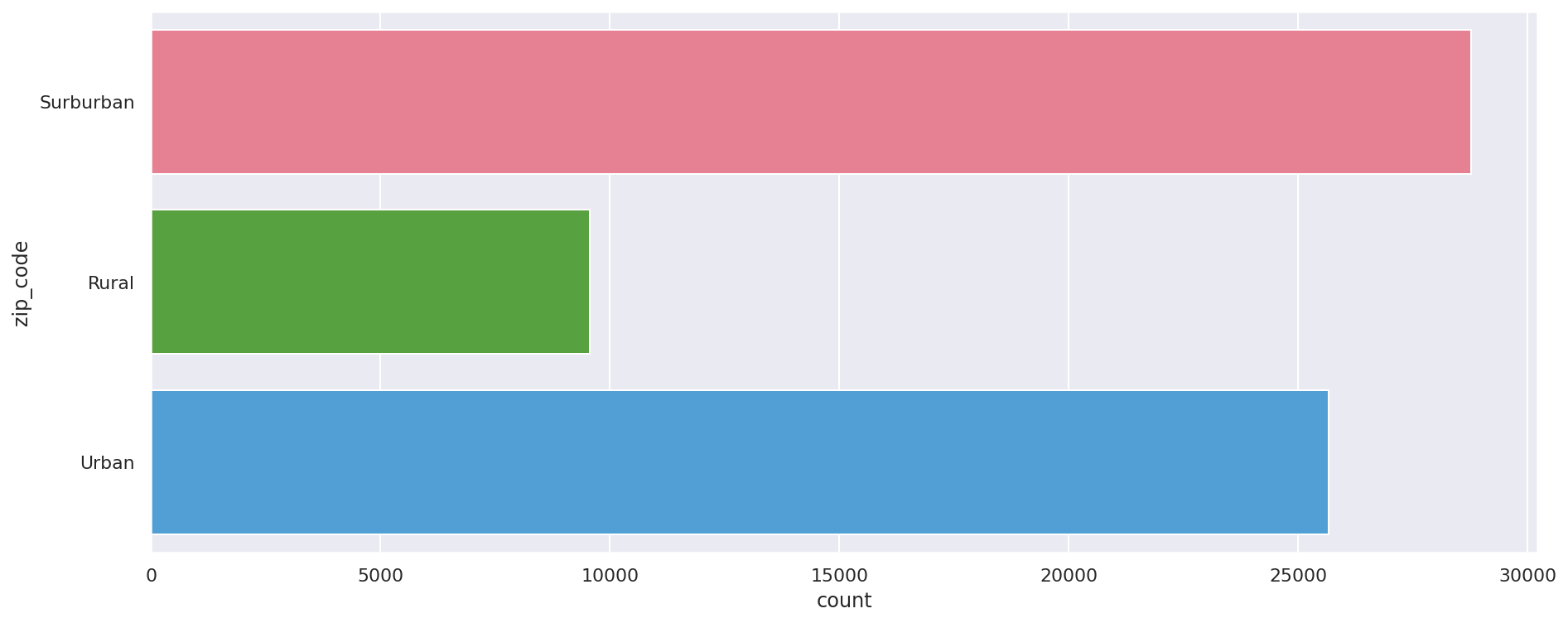### Strip plots

Strip plots are essentially scatterplots for categorical variables. They can be used in several ways but require an `x` (categorical) and `y` (numeric) value as a minimum requirement. Here’s a strip plot showing the historical purchases by customers in the three channels. Note that the multichannel data starts above zero, because you have to have shopped in two channels to be multichannel.

``````sns.stripplot(x="channel", y="history", data=df, palette="husl")
``````
``````<matplotlib.axes._subplots.AxesSubplot at 0x7fb9f85e7eb0>
``````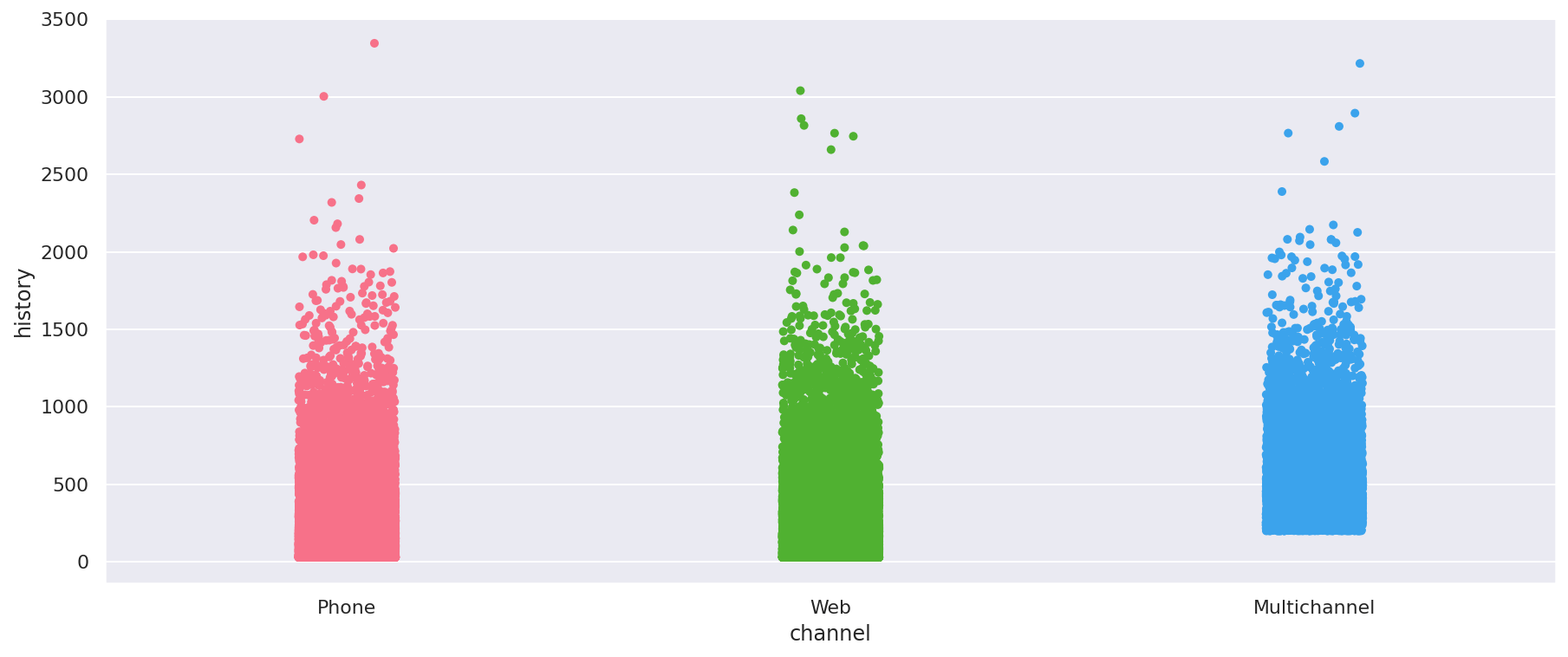You can also add a `hue` to strip plots to show an additional categorical variable. In the example below, I’ve added `conversion` as a `hue`.

``````sns.stripplot(x="history", y="channel", hue="conversion", data=df, palette="husl")
``````
``````<matplotlib.axes._subplots.AxesSubplot at 0x7fb9f83f3700>
``````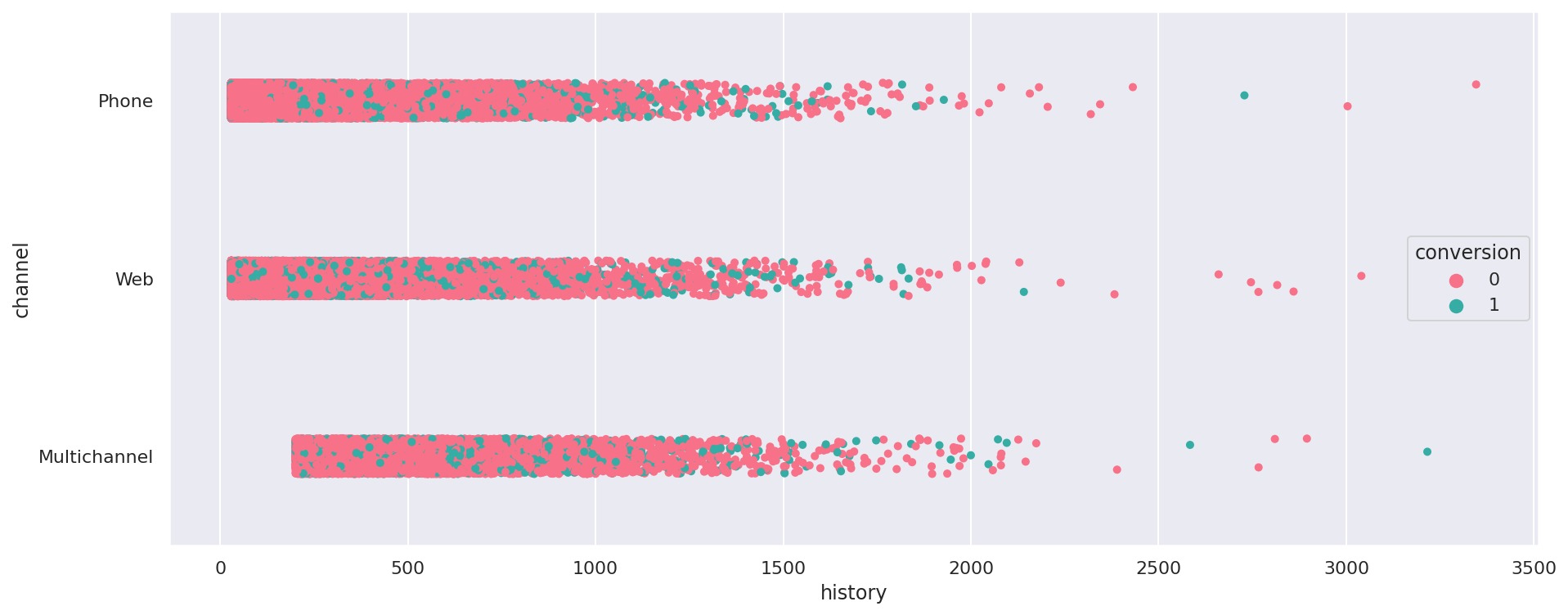### Box plots

In their standard form (see below) box plots, or box and whisker diagrams, are typically used to show the distribution of values within a column. They’re a great way to see where most of the data lies, and for identifying outliers.

``````sns.boxplot(x=df["history"])
``````
``````<matplotlib.axes._subplots.AxesSubplot at 0x7fb9f2096eb0>
``````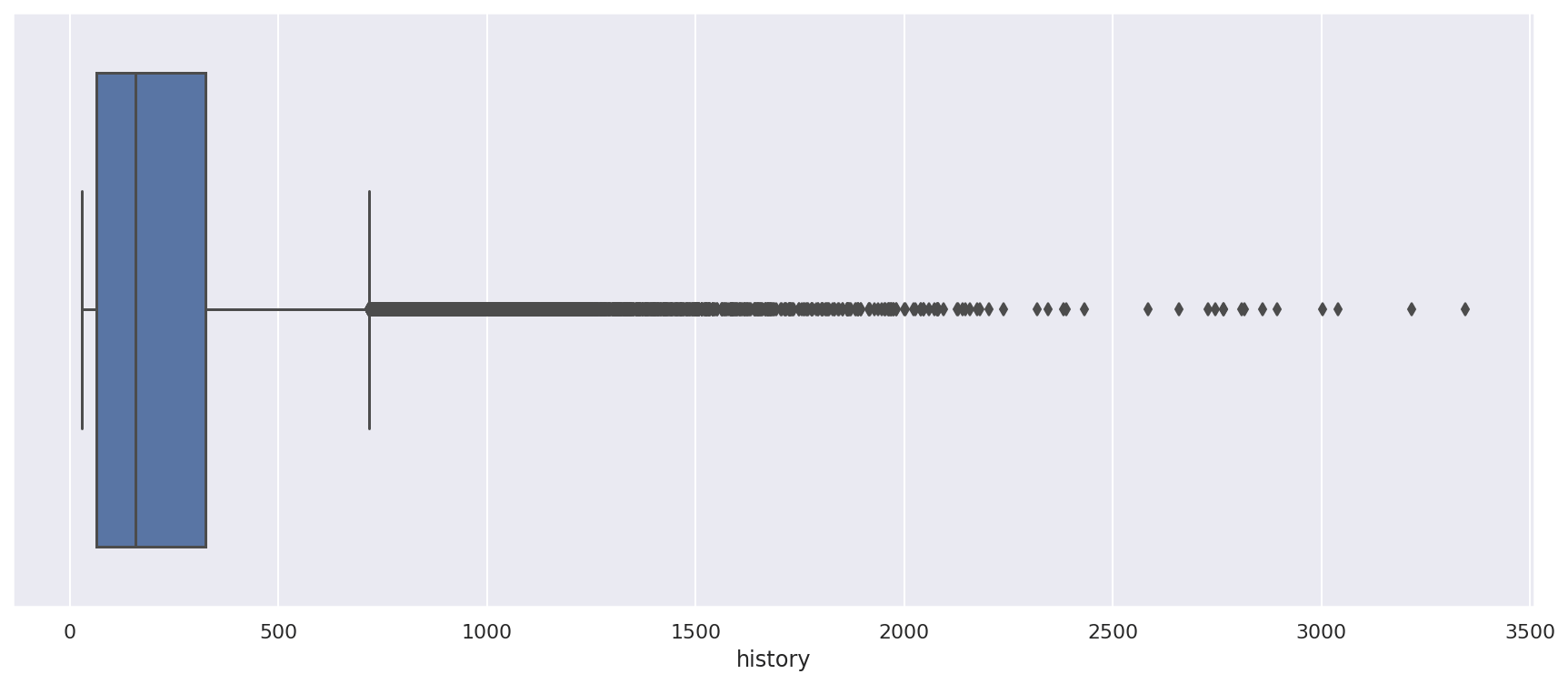However, boxplots are particularly useful for comparing the spread of categorical data. Here’s the `history` column for each `channel` in the data set. It clearly shows that online and telephone customers are very similar, but multichannel customers have a higher value.

``````sns.boxplot(x="channel", y="history", data=df, palette="husl")
``````
``````<matplotlib.axes._subplots.AxesSubplot at 0x7fb9f213eca0>
``````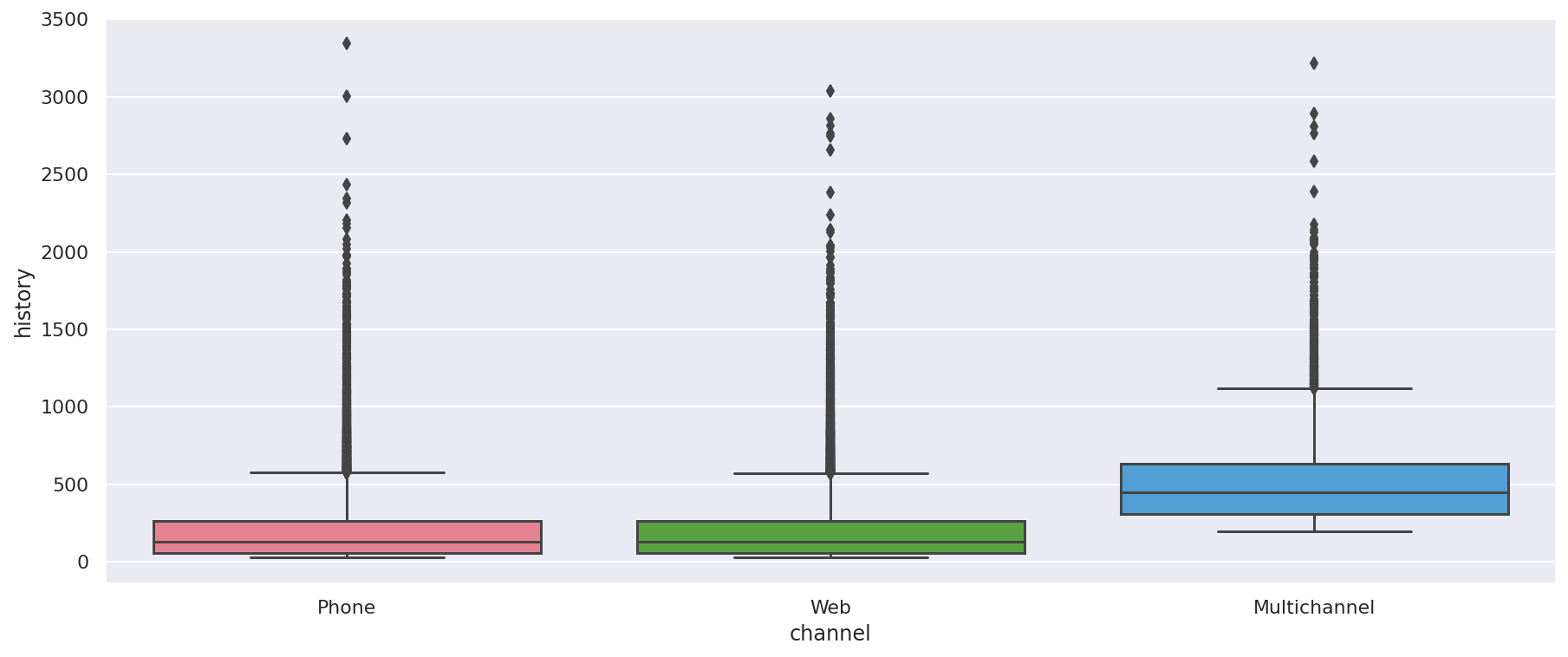You can also pass in an additional `hue` argument to boxplots. Here’s the above data with an additional `hue` argument which splits the channel boxplots up by the offer type. As you can see, the customers were balanced carefully across the offer types to try and ensure the marketing campaign generated valid results.

``````sns.boxplot(x="channel", y="history", hue="offer", data=df, palette="husl")
``````
``````<matplotlib.axes._subplots.AxesSubplot at 0x7fb9f82c5af0>
``````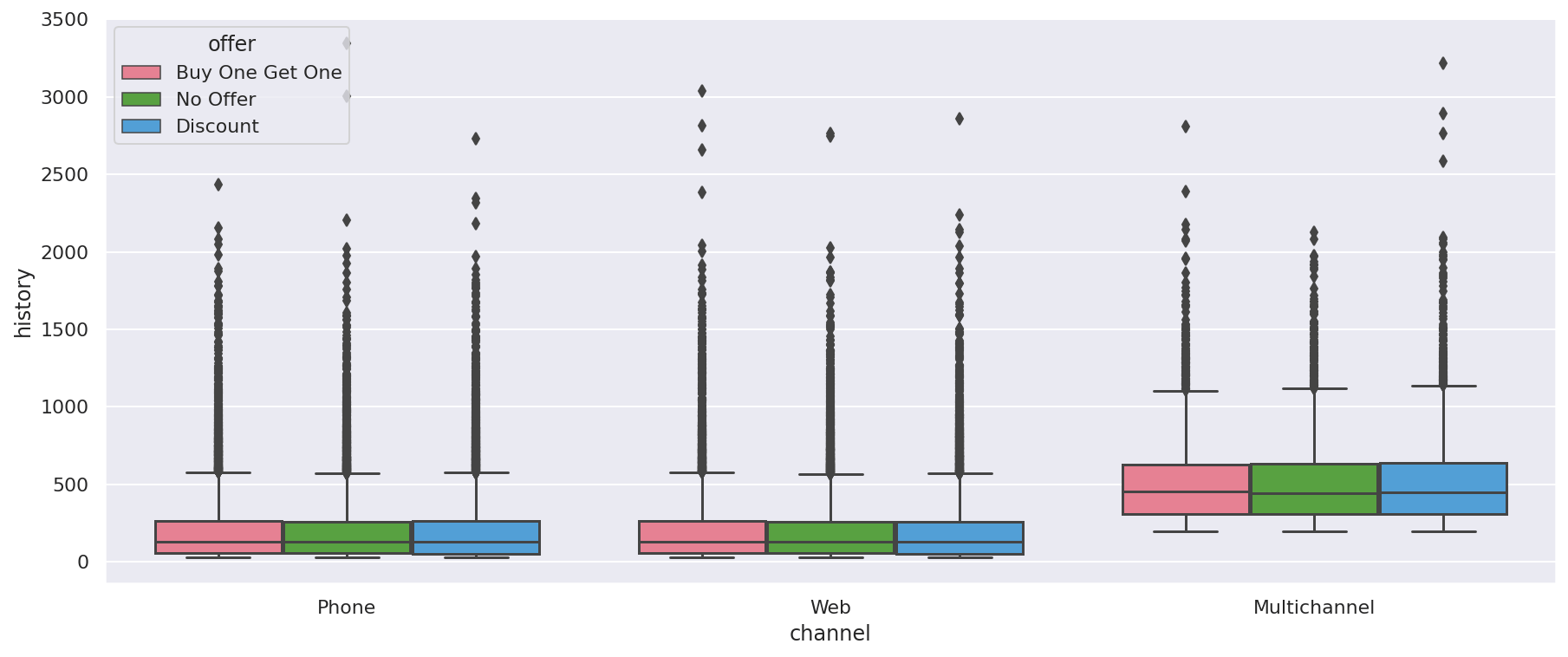### Violin plots

Violin plots are much like box plots, as they show the quantitative distribution of data. The width of the violinplot denotes the number of values in that area, so they can be a bit more intuitive than boxplots to interpret.

``````sns.violinplot(x=df["history"], palette="husl")
``````
``````<matplotlib.axes._subplots.AxesSubplot at 0x7fb9f3abdc70>
``````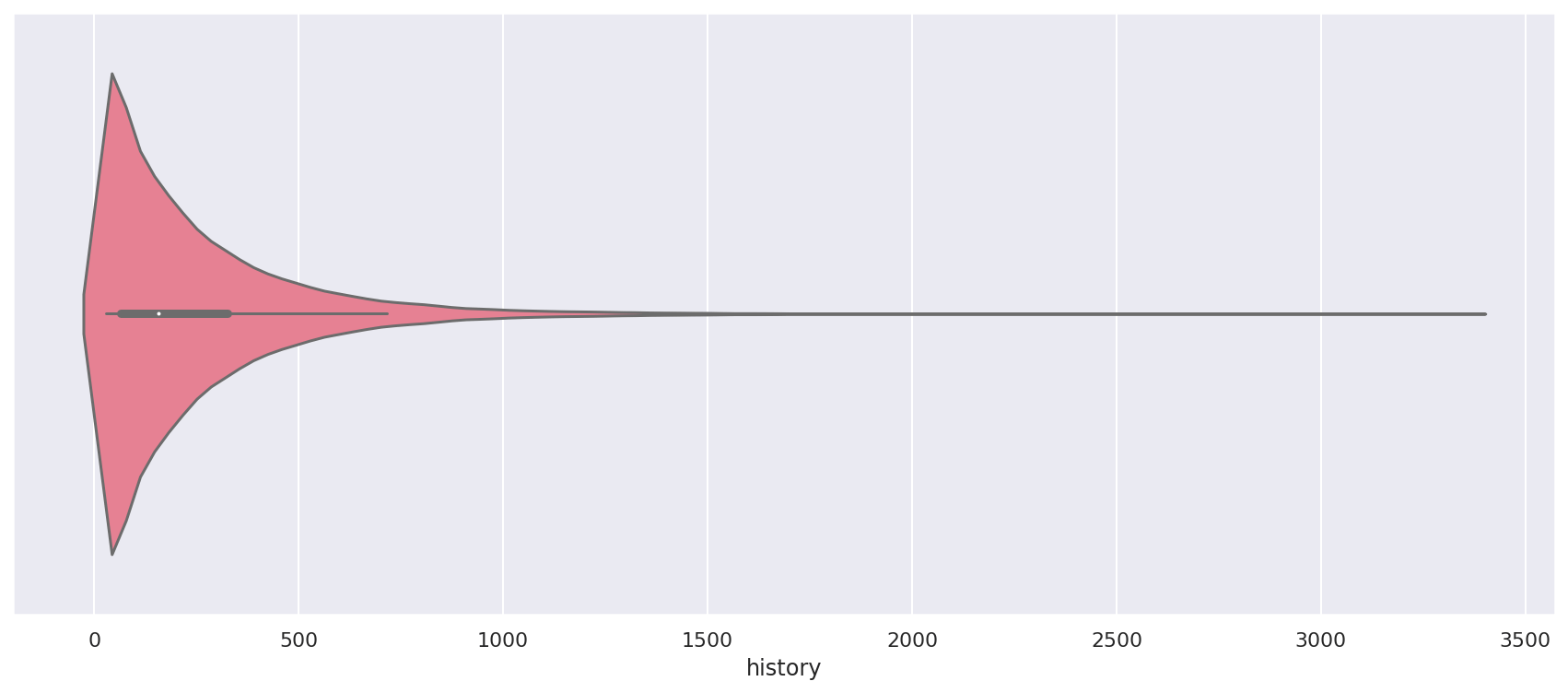As with the boxplot, it’s when you add categorical columns to violin plots that they really become useful. Here’s a plot showing the spread of `recency` data for each of the channels. This shows that the online and telephone channels are almost identical, but that the multichannel customers have a much higher recency.

``````sns.violinplot(x="channel", y="recency", data=df, palette="husl")
``````
``````<matplotlib.axes._subplots.AxesSubplot at 0x7fb9f21e6c70>
``````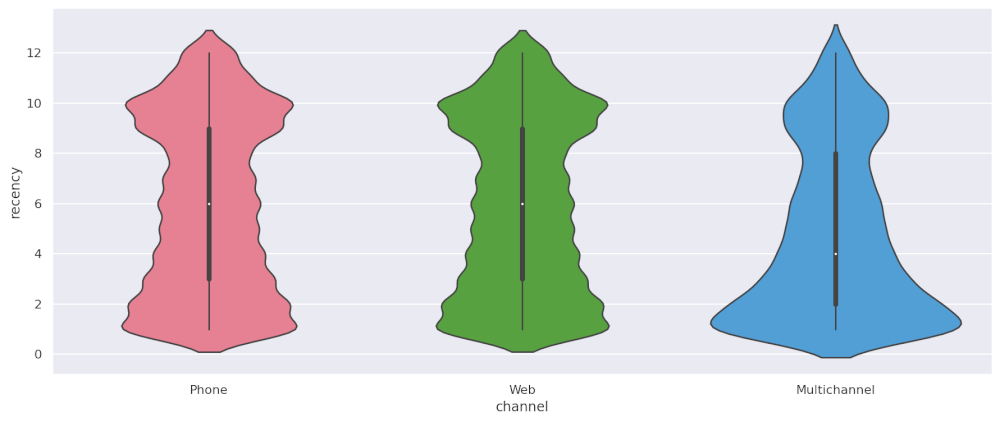#### Split violin plots

With violin plots, adding the additional `hue` argument defining an additional categorical column creates something called a split violin plot. The one below shows the recency by channel, but splits the violin plot according to whether customers purchased or didn’t. Recency seems to make a big difference.

``````sns.violinplot(x="channel", y="recency", hue="conversion", data=df, palette="husl", split=True)
``````
``````<matplotlib.axes._subplots.AxesSubplot at 0x7fb9f21d8a30>
``````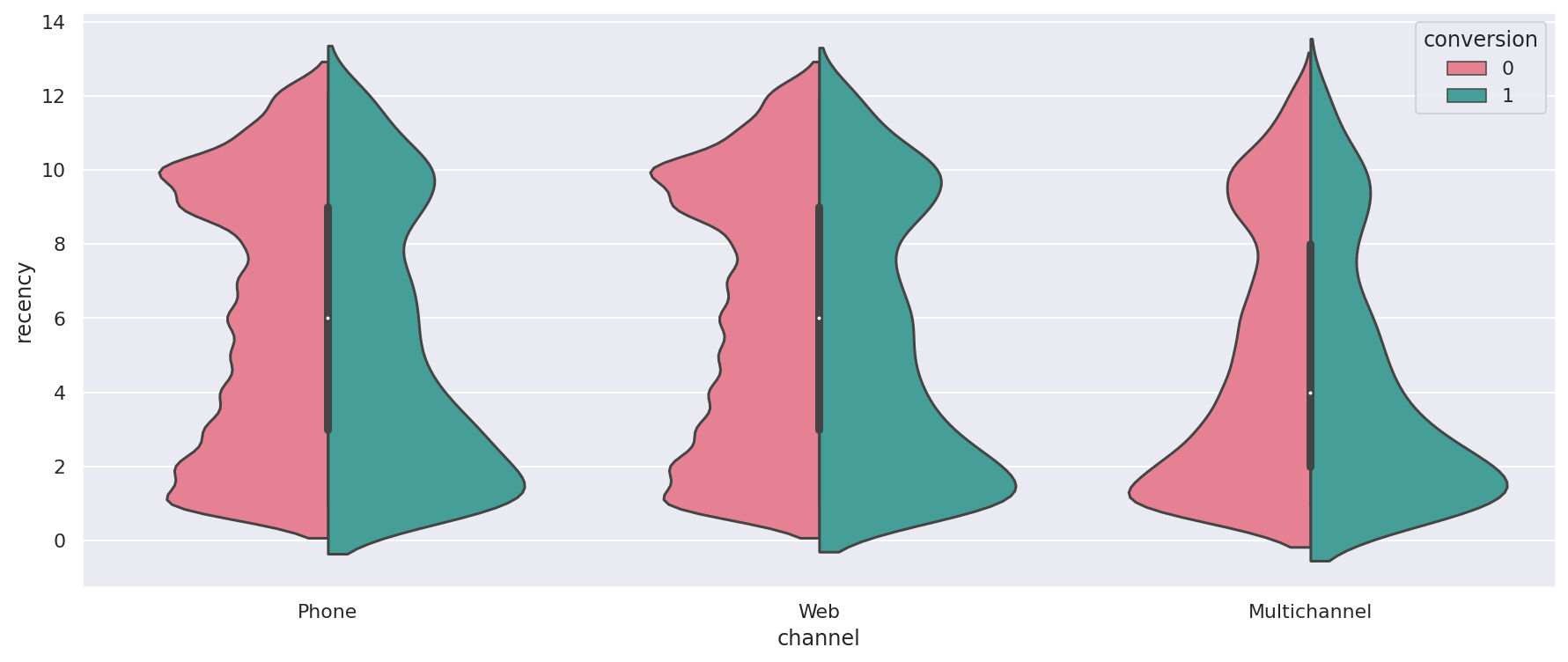### Boxen plots

Finally, the boxen plot or letter value plot. These are kind of like a cross between a box plot and a histogram, as it shows data binned into quantiles. Again, by default they show the spread of values in a single column, but can be very useful when an additional column is added.

``````sns.boxenplot(x=df["recency"], palette="husl")
``````
``````<matplotlib.axes._subplots.AxesSubplot at 0x7fb9f21aadc0>
``````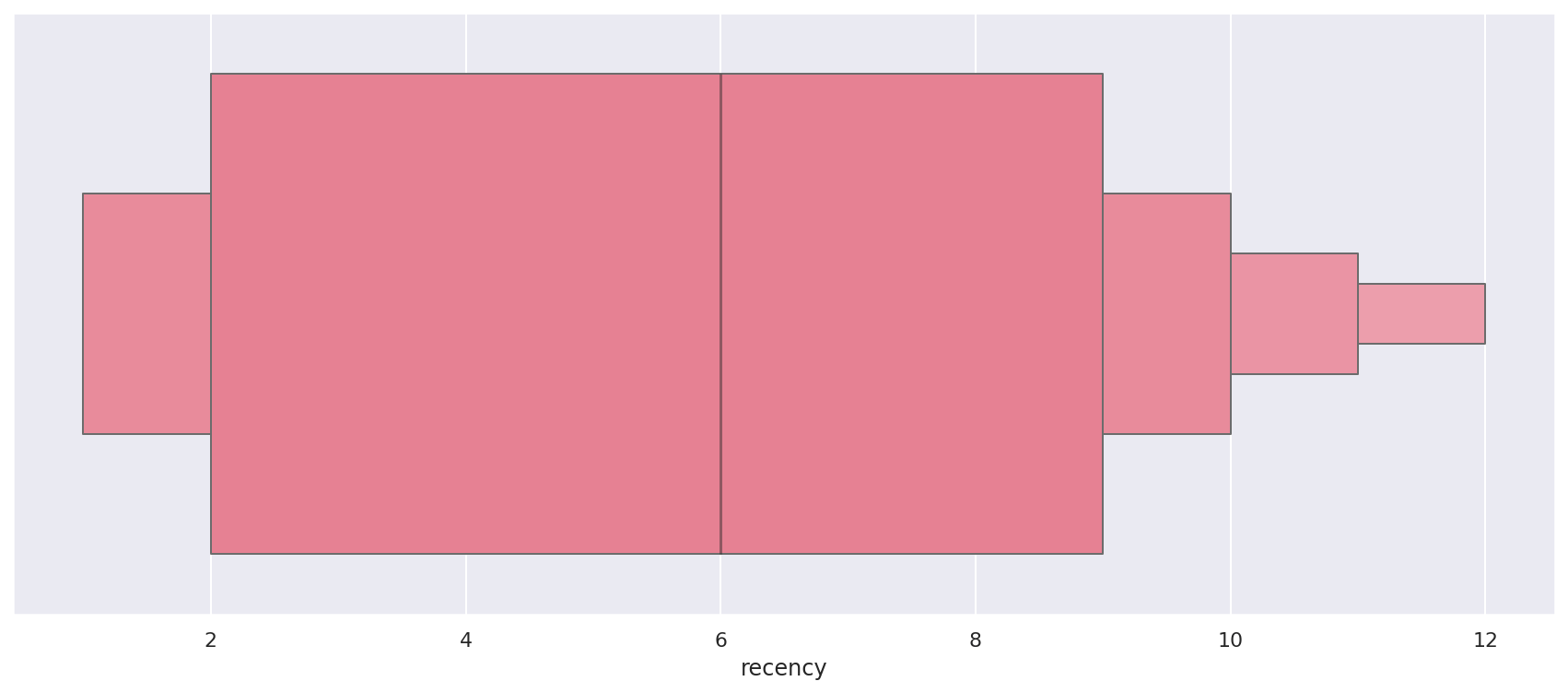Here’s a boxen plot for the channel and recency columns again, showing the nearly identical shapes of the phone and web channels, and the different shape of the multichannel customers, where more of the customers are more recent.

``````sns.boxenplot(x="channel", y="recency", data=df, palette="husl")
``````
``````<matplotlib.axes._subplots.AxesSubplot at 0x7fb9f1f4eac0>
``````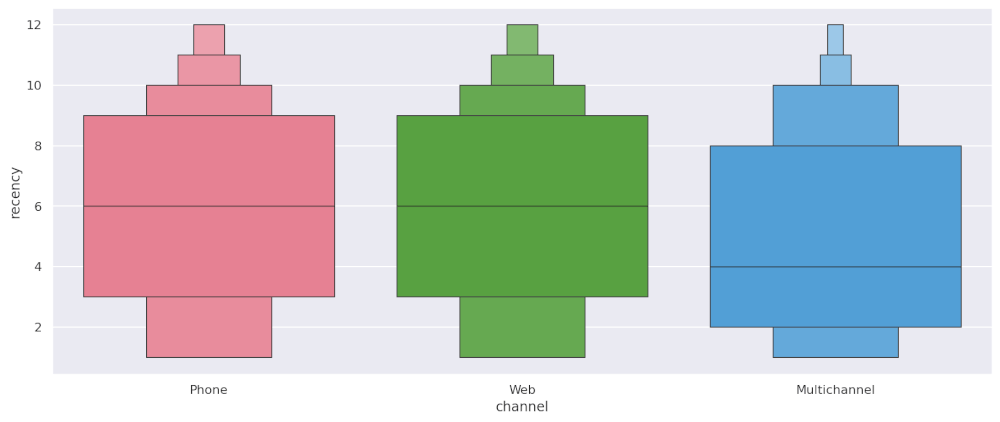As with the other plots, an additional `hue` argument can also be included to show another categorical variable. Like the box plot, these are also a good way to visualise the presence of outliers that can often impact model performance.

``````sns.boxenplot(x="channel", y="history", hue="zip_code", data=df, palette="husl")
``````
``````<matplotlib.axes._subplots.AxesSubplot at 0x7fb9eab7a850>
``````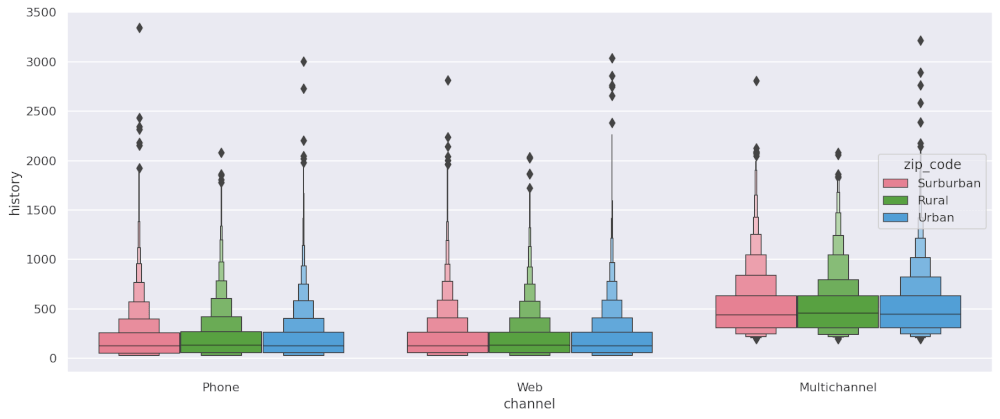Matt Clarke, Sunday, March 07, 2021

Matt Clarke Matt is an Ecommerce and Marketing Director who uses data science to help in his work. Matt has a Master's degree in Internet Retailing (plus two other Master's degrees in different fields) and specialises in the technical side of ecommerce and marketing.